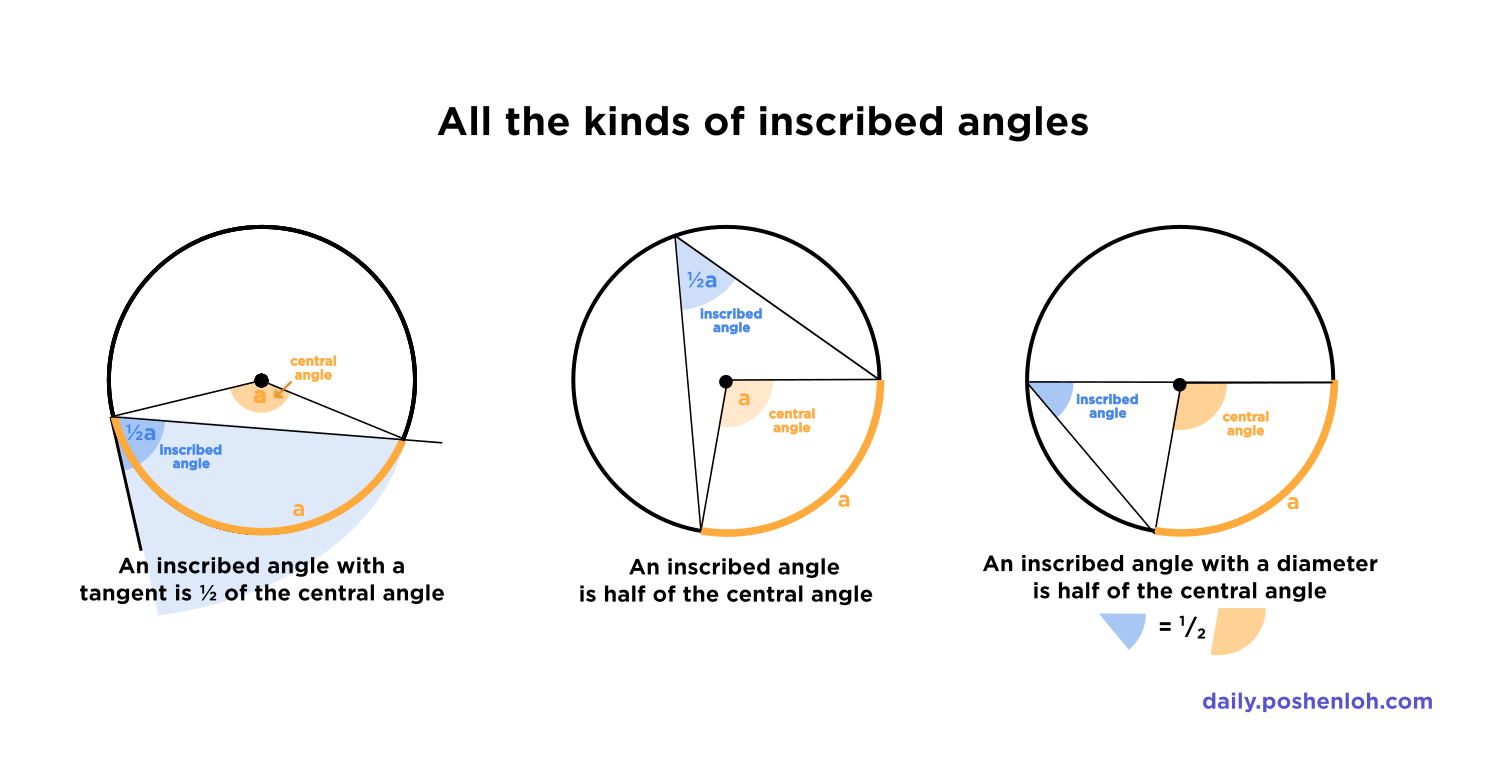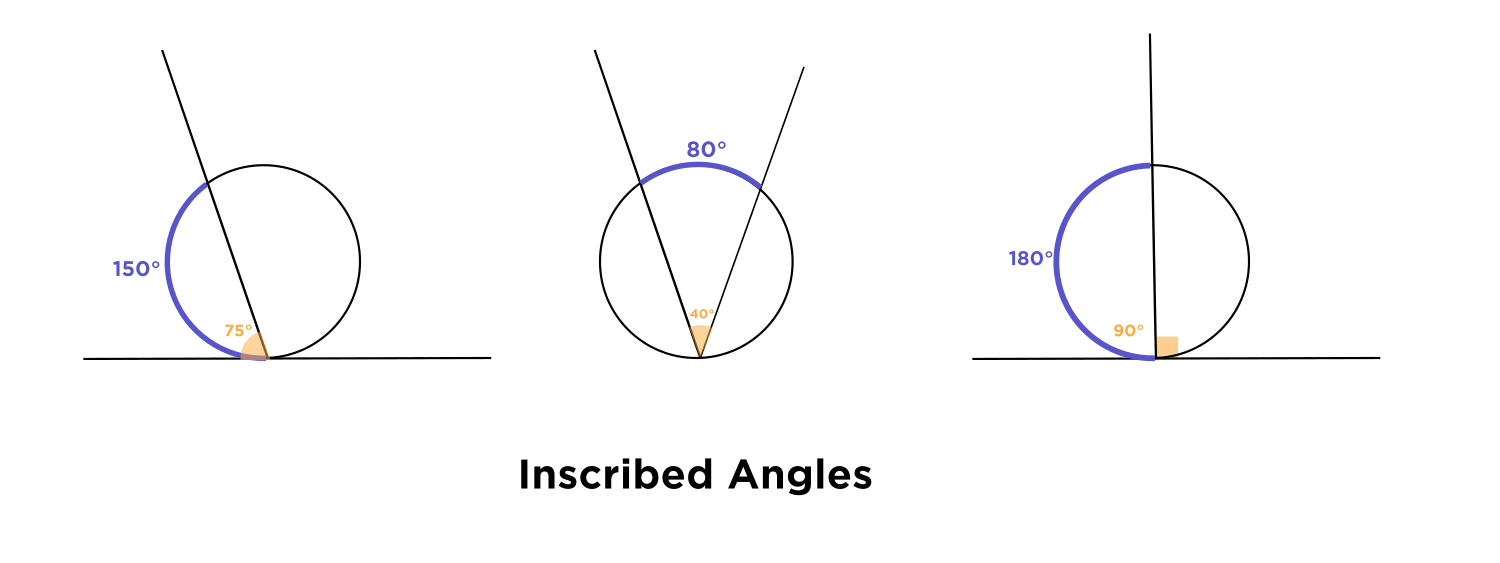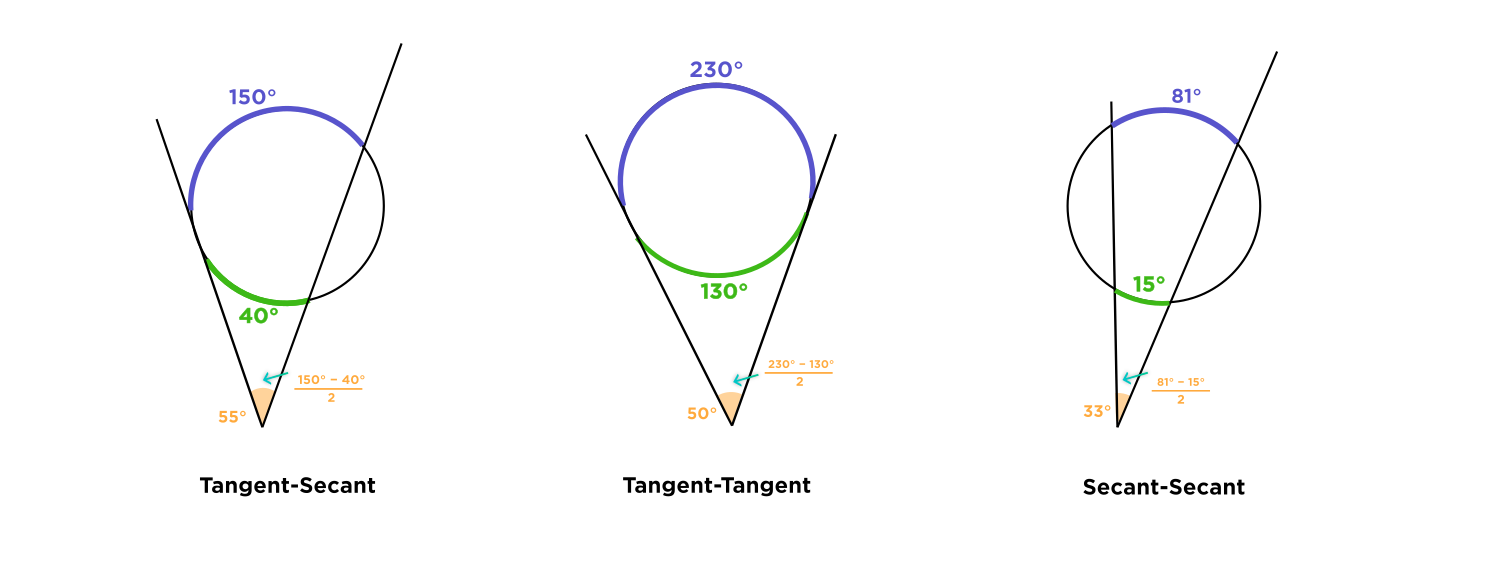# Visuals for Inscribed Angles

• ## Type 1:

The vertex (corner) of the angle is lying on the circle's circumference. This is called an inscribed angle, and to find the measure of the angle, take the measure of the arc that it inscribes (opens up to), and divide by $$2.$$Type 2: The vertex (corner) of the angle lies outside the circle, but the lines that make the angle intersect the circle. The lines of the angle may be tangent (touching the circle at only one point) or secant (intersecting the circle in two points). Either way, the formula for the measure of the angle is the same:

$$\text{ angle } = \frac{ \text{larger arc measure} - \text{ smaller arc measure} }{2}$$Prof. Loh proved this neat fact in the Day 10 Your Turn lessons!Now, I hope you have fun and know what to do whenever you see an inscribed angle!